# Colleges with the lowest SAT scores in South Carolina

Top 10 colleges in South Carolina with the lowest SAT scores
Looking for the colleges with the lowest SAT scores in South Carolina? Well you're in luck! We've compiled a national college database and have created a list of the top 10 universities with the lowest SAT scores in South Carolina below. If you are not a good test taker or worried about your test scores, this list is for you. These are the schools whose applicants had the lowest average SAT scores in South Carolina, which means that you can get into these colleges with a lower SAT score. We also include each college's ACT scores and acceptance rate so that you can see where you would have the easiest time getting in. Read on to find out more.

## South Carolina State University SAT scores

The average SAT score for South Carolina State University is 940.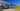The average SAT score of 940 breaks down into:

• SAT math: 460

The average ACT score for South Carolina State University is 16 and their acceptance rate is 53.2%.

## Francis Marion University SAT scores

The average SAT score for Francis Marion University is 990.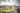The average SAT score of 990 breaks down into:

• SAT math: 490

The average ACT score for Francis Marion University is 20 and their acceptance rate is 60%.

## Coker College SAT scores

The average SAT score for Coker College is 1020.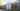The average SAT score of 1020 breaks down into:

• SAT math: 500

The average ACT score for Coker College is 20 and their acceptance rate is 61.7%.

## Columbia College South Carolina SAT scores

The average SAT score for Columbia College South Carolina is 1020.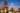The average SAT score of 1020 breaks down into:

• SAT math: 500

The average ACT score for Columbia College South Carolina is 20 and their acceptance rate is 86.8%.

## University of South Carolina Beaufort SAT scores

The average SAT score for University of South Carolina Beaufort is 1020.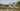The average SAT score of 1020 breaks down into:

• SAT math: 500

The average ACT score for University of South Carolina Beaufort is 20 and their acceptance rate is 62.7%.

## Newberry College SAT scores

The average SAT score for Newberry College is 1030.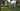The average SAT score of 1030 breaks down into:

• SAT math: 510

The average ACT score for Newberry College is 20 and their acceptance rate is 81.9%.

## University of South Carolina Upstate SAT scores

The average SAT score for University of South Carolina Upstate is 1030.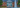The average SAT score of 1030 breaks down into:

• SAT math: 510

The average ACT score for University of South Carolina Upstate is 20 and their acceptance rate is 56%.

## Columbia International University SAT scores

The average SAT score for Columbia International University is 1040.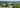The average SAT score of 1040 breaks down into:

• SAT math: 510

The average ACT score for Columbia International University is 20 and their acceptance rate is 33.4%.

## Limestone College SAT scores

The average SAT score for Limestone College is 1050.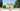The average SAT score of 1050 breaks down into:

• SAT math: 520

The average ACT score for Limestone College is 20 and their acceptance rate is 44.2%.

## University of South Carolina Aiken SAT scores

The average SAT score for University of South Carolina Aiken is 1056.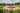The average SAT score of 1056 breaks down into:

• SAT math: 520